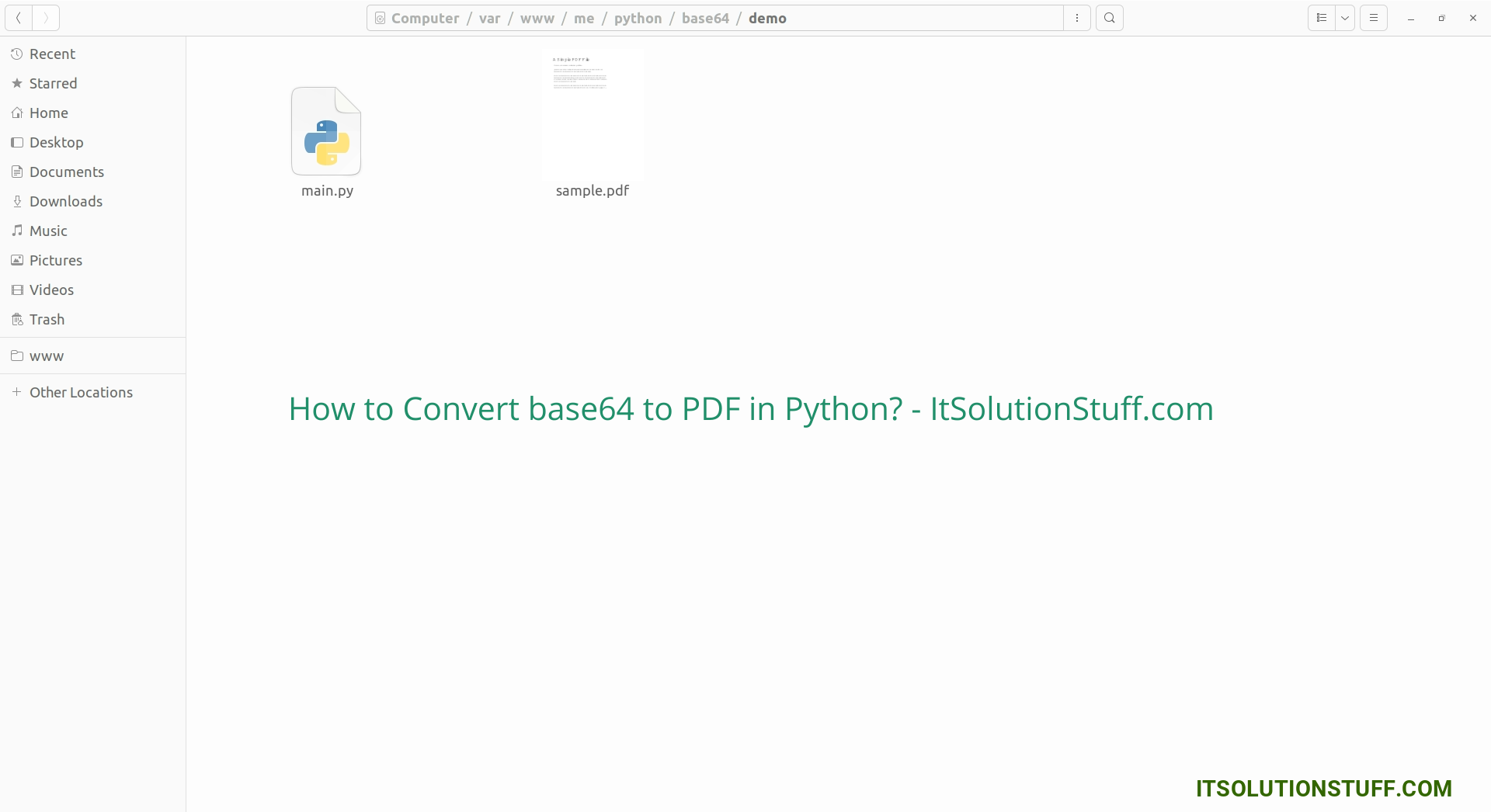# How to Convert base64 to PDF in Python?

By Hardik Savani October 30, 2023 Category : Python

Hello Guys,

Now, let's see post of how to convert base64 to pdf in python. I explained simply about python convert base64 to pdf. Iâ€™m going to show you about how to convert base64 string to pdf in python. This example will help you how to decode base64 string to pdf in python.

If you are looking to convert base64 string to an pdf in python then I will help you with that. I will give you a very simple example, we will take one variable "pdfData" with base64 string and then convert it into the pdf. we will use base64 library. So, without further ado, let's see simple examples: You can use these examples with python3 (Python 3) version.

Example:

main.py

```# Import base64 Module
import base64

# PDF Base64 String
pdfData = "JVBERi0xLjMNCiXi48/TDQoNCjEgMCBvYmoNCjw8DQovVHlw...";

# Decode base64 String Data
decodedData = base64.b64decode((pdfData))

# Write PDF from Base64 File
pdfFile = open('sample.pdf', 'wb')
pdfFile.write(decodedData)
pdfFile.close()
```

Output: